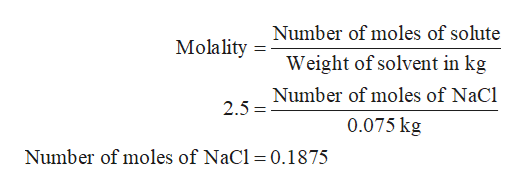# If 500. g of water is added to a 75 g of a 2.5 molality NaCl solution, what is the mass percent of NaCl in the diluted solution?

Question
317 views

If 500. g of water is added to a 75 g of a 2.5 molality NaCl solution, what is the mass percent of NaCl in the diluted solution?

check_circle

Step 1

Given:

Mass of water is 500 g.

Mass of NaCl is 75 g.

Molality of NaCl is 2.5

Step 2

It is known, 1 g = 0.001 kg

Therefore, mass of NaCl in kg is 0.075 kg.

The mathematical expression of molality is,

Step 3

Substitute the given value of molality and weight...help_outlineImage TranscriptioncloseNumber of moles of solute Molality = Weight of solvent in kg Number of moles of NaCl 2.5 0.075 kg Number of moles of NaCl = 0.1875 fullscreen

### Want to see the full answer?

See Solution

#### Want to see this answer and more?

Solutions are written by subject experts who are available 24/7. Questions are typically answered within 1 hour.*

See Solution
*Response times may vary by subject and question.
Tagged in

### Solutions